﻿ 基于图染色的铁路调车场调车问题研究

# 基于图染色的铁路调车场调车问题研究Research on Railway Shunting Problem Based on Graph Coloring

Abstract: This paper mainly studies the shunting problem of railway shunting yard. Compared with the existing research, this paper considers both the number limit and the capacity limit of the shearing yard, which is more in line with the actual situation. Firstly, we transform the shunting problem of the shunting yard with the limitation of the number of channels and the limitation of the capacity of channels into a special pattern dyeing problem. Then, a greedy algorithm is designed for this problem, and the numerical experiment shows that the algorithm is suitable for practical application.

1. 引言

1.1. 背景介绍

1.2. 相关工作

Futhner  的方法是最古老的排序方法之一，该方法在不考虑车辆进站顺序的情况下，为出站的车辆序列构造分类表。随着时间的推移，类似的基于规则的重新安排车辆的方法被开发出来并应用于实践。德国Schweizer Bundesbahnen的运兵学研究组   将基于规则的重新安排车辆的方法开发出来并应用于实践，其中最著名的是同步三角形，或几何图形。基于规则的启发式方法的优点是简单和透明，在不考虑车辆进站顺序的条件下，经验丰富的工作人员在通常情况下可以进行有效调度。Cicerone等  首先考虑了分流的概念。将研究重点放在分流场classifification problems (CP) (通常称为分类碗)中对列车编组和列车分类进行建模的分类问题，即研究将进站车辆分配给出站列车的方案。讨论CP的离线变体，其中所有相关信息都是已知的，并且不允车辆同时到达。在任何变体中，对于进站的车辆存在三种移动过程：从分类碗中的入站股道移动到分拣股道(i-t-move)；从分拣股道移动到出站股道(t-o-move)；从分拣股道移动到另一个分拣股道(t-t-moves)。如果车辆不允许t-t-move的调度，则为单级变体，否则为多阶段的变化。在此基础上，对不限制股道数量或容量的分类碗进行了研究。

1.3. 本文贡献

1) 将带有股道数量与股道容量约束条件的调车场调车问题转化为二部图上的边染色问题。已有研究将调车场调车问题转化为图染色问题进行研究时，会假设可使用的股道数量是无限的，主要优化目标为使用尽可能少的股道数。而我们所考虑的问题中股道的数量与容量都有限制，更加符合实际情况。

2) 设计了关于上述图染色问题模型的贪婪算法，数值实验表明我们所设计的算法能够快速有效地求解上述模型，得到面向需求的铁路股道车辆调度优化方案，从而表明本文所提出的铁路股道车辆调度方法可以应用于实际的铁路股道车辆调度工作中。

2. 模型

2.1. 问题背景Figure 1. Schematic diagram of railway station track infrastructure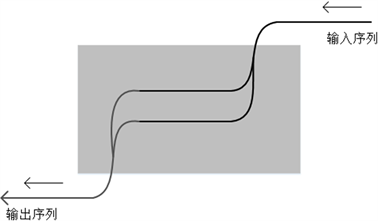Figure 2. Diagram of single in single out scheduling mode

2.2. 调车场调车问题

T：铁路车站内股道的数量，且按照顺序依次代表股道的编号；

R：车站内股道的容量，每条股道的容量均相同；

c：列车上搭载车辆的数量 $0，所有列车搭载车辆的数量均为c；

d：到站车辆的目的地车站种类数量， $d\in {N}^{*}$$d>m$

D：目的地车站集合 $D=\left\{1,2,\cdots ,d\right\}$，每个车辆有且只有一个目的地车站；

${d}_{j}$ ：表示车辆j的目的地车站， ${d}_{j}\in D$

V：车辆编组计划

1) 车辆目的地集合 $D=\left\{1,2,\cdots ,d\right\}$，每个车辆有且只有一个目的地车站。目的地车站的数量d远大于股道数量T。

2) 车辆编组计划 $V=\left\{{V}_{1},{V}_{2},\cdots ,{V}_{k}\right\}$${V}_{i}={d}_{1}{d}_{2}\cdots {d}_{j}$$\forall {d}_{j}\in D\left(j=1,2,3,\cdots ,c\right)$ 是一个长度为n的数组，数组中的元素都属于目的地集合D。表示对于输入的n个车辆编组成新列车的计划。

1、股道数量约束，同一时间可使用的股道数不超过T；

2、股道容量约束，任意时刻一条股道上停放的车辆数不超过R；

3、不容许车辆在股道之间进行移动。

2.3. 车辆编组染色问题

$dest=U+V\to D$

$\stackrel{¯}{C}=C\cup \left\{\text{NUL}\right\}$

$\phi \left({S}_{k}\right)=\left\{\begin{array}{l}1,\text{\hspace{0.17em}}\text{\hspace{0.17em}}\text{\hspace{0.17em}}\text{if}\text{\hspace{0.17em}}{S}_{k}\ne \phi \\ 0,\text{\hspace{0.17em}}\text{\hspace{0.17em}}\text{\hspace{0.17em}}\text{\hspace{0.17em}}\text{\hspace{0.17em}}\text{\hspace{0.17em}}\text{else}\end{array},\text{\hspace{0.17em}}\text{\hspace{0.17em}}\text{\hspace{0.17em}}\text{\hspace{0.17em}}\text{\hspace{0.17em}}\forall k=1,2,\cdots$

$\text{color}\left(G\right)={\sum }_{k=1}^{\infty }\phi \left( S k \right)$

${{u}^{\prime }}_{i}<{u}_{i}$

${{v}^{\prime }}_{j}<{v}_{j}$

$\delta \left(\left({{u}^{\prime }}_{i},{{v}^{\prime }}_{j}\right),\left({u}_{i},{v}_{j}\right)\right)=\left\{\begin{array}{l}1,\text{\hspace{0.17em}}\text{\hspace{0.17em}}\text{\hspace{0.17em}}\text{if}\text{\hspace{0.17em}}{i}^{\prime }

$\text{color}\left(\left({u}_{i},{v}_{j}\right)\right)\ne \text{NUL}$$\text{color}\left(\left({u}_{i},{v}_{j}\right)\right)\ne k$

$\text{reside}\left({S}_{k},\left({u}_{i},{v}_{j}\right)\right)=\underset{\left({{u}^{\prime }}_{i},{{v}^{\prime }}_{j}\right)\in {S}_{k}}{\sum }\delta \left(\left({u}_{i},{v}_{j}\right),\left({{u}^{\prime }}_{i},{{v}^{\prime }}_{j}\right)\right)$

$\text{reside}\left({S}_{k}\right)=\underset{\begin{array}{c}\text{color}\left(\left({u}_{i},{v}_{j}\right)\right)\ne \text{NUL}\\ \text{color}\left(\left({u}_{i},{v}_{j}\right)\right)\ne k\end{array}}{\mathrm{max}}\left\{\text{reside}\left({S}_{k},\left({u}_{i},{v}_{j}\right)\right)\right\}$

1) 染色color是无冲突的；

2) 边集 ${\sum }_{k=1}^{\infty }{S}_{k}$ 是二部图G的一个完美匹配；

3) 函数color(G)如定义3所定义， $\text{color}\left(G\right)\le T$

4) 函数如定义5所定义， $\text{reside}\left({S}_{k}\right)\le R$$k=1,2,\cdots$

${f}_{x}\left({u}_{i}\right)=\left\{\begin{array}{l}{{u}^{\prime }}_{i},\text{\hspace{0.17em}}\text{\hspace{0.17em}}\text{\hspace{0.17em}}\text{\hspace{0.17em}}\text{if}\text{\hspace{0.17em}}1\le i 其中 $1\le x

${f}_{x}\left({v}_{j}\right)={{v}^{\prime }}_{j}$$j=1,2,\cdots ,n.$

$dest\left({u}_{i}\right)=I\left[i\right],\text{\hspace{0.17em}}\text{\hspace{0.17em}}i=1,2,\cdots ,n$

$dest\left({v}_{j}\right)=O\left[j\right],\text{\hspace{0.17em}}\text{\hspace{0.17em}}j=1,2,\cdots ,n$

3. 算法

3.1. 算法设计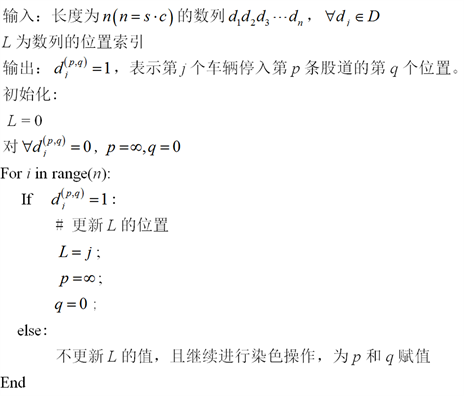3.2. 数值实验

4. 结论与展望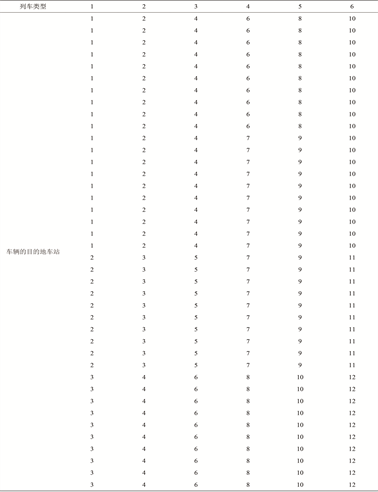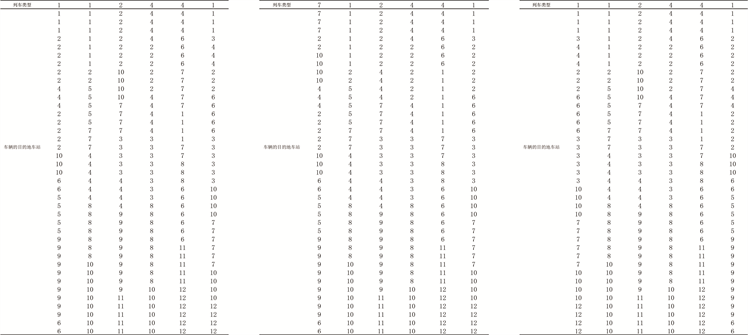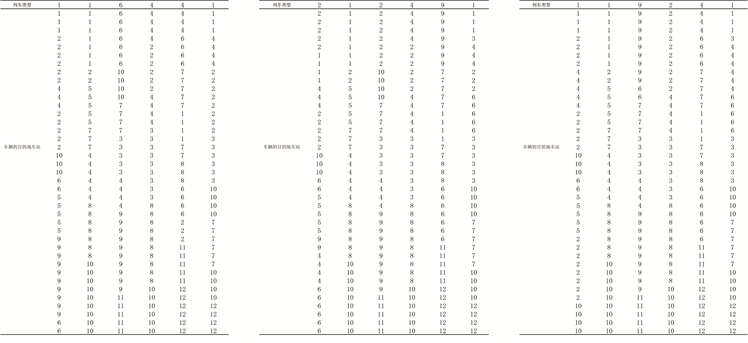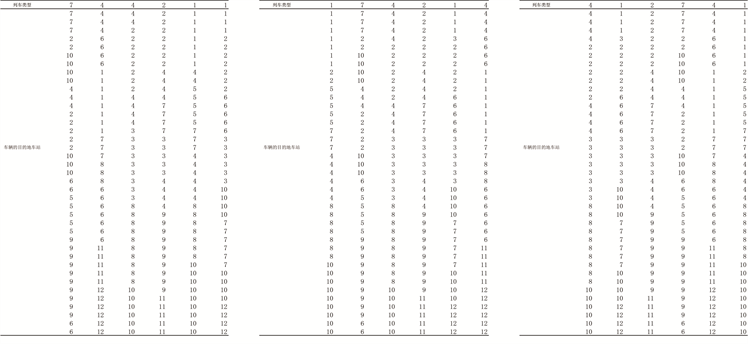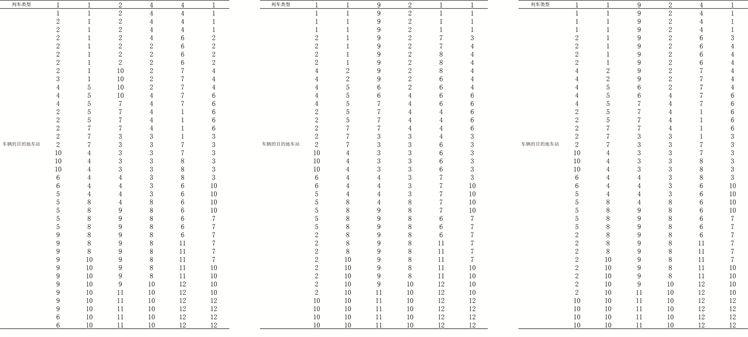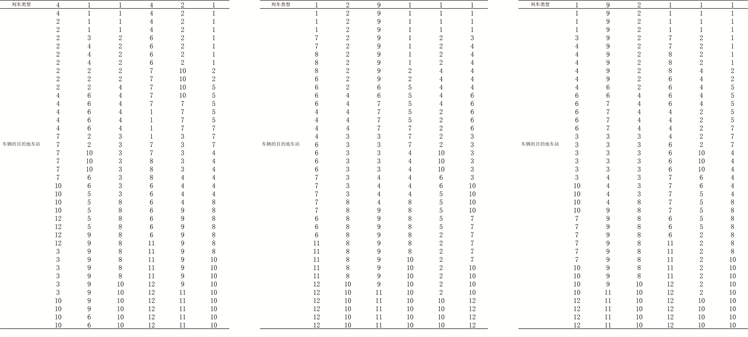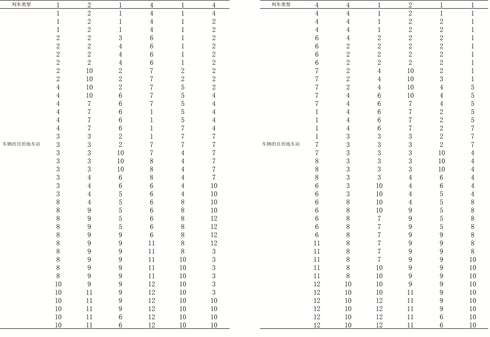Nemani, A.K. and Ahuja, R.K. (2011) OR Models in Freight Railroad Industry. In: Cochran, J.J., Cox, L.A., Keskinocak, P., Kharoufeh, J.P. and Smith, J.C., Eds., Wiley Encyclopedia of Operations Research and Management Science, Wiley, London, 182.
https://doi.org/10.1002/9780470400531.eorms0622

 赵星宇, 丁世飞. 深度强化学习研究综述[J]. 计算机科学, 2018, 45(7): 7-12.

 Levine, S., Pastor, P., Krizhevsky, A., et al. (2016) Learning Hand-Eye Coordination for Robotic Grasping with Large-Scale Data Collection. In: International Symposium on Experimental Robotics, Springer, Cham, 173-184.
https://doi.org/10.1007/978-3-319-50115-4_16

 Zhang, M., Mccarthy, Z., Finn, C., et al. (2016) Learning Deep Neural Network Policies with Continuous Memory States. Proceedings of the International Conference on Robotics and Automation, Stockholm, 16-21 May 2016, 520-527.
https://doi.org/10.1109/ICRA.2016.7487174

 Lenz, S., Finn, C., Darrell, T., et al. (2016) End-to-End Training of Deep Visuomotor Policies. Journal of Machine Learning Research, 17, 1-40.

 Lenz, I., Knepper, R. and Saxena, A. (2015) Deepmpc: Learning Deep Latent Features for Model Predictive Control. Proceedings of the Robotics Science and Systems, Rome, 13-17 July 2015, 201-209.

 Satija, H. and Pineau, J. (2016) Simultaneous Machine Translation Using Deep Reinforcement Learning. Proceedings of the Workshops of International Conference on Machine Learning, New York, 20-22 June 2016, 110-119.

 Oh, J., Guo, X., Lee, H., et al. (2015) Action-Conditional Video Prediction Using Deep Networks in Atari Games. Annual Conference on Neural Information Processing Systems, Montreal, 7-12 December 2015, 2863-2871.

 Guo, H. (2015) Generating Text with Deep Reinforcement Learning. Proceedings of the Workshops of Advances in Neural Information Processing Systems, Montreal, 1-9.

 马亮, 张晓霞, 郭进. 基于启发式回溯算法的铁路编组站调车场股道活用研究[J]. 铁道学报, 2016, 38(8): 16-22.

 Boysen, N., Emde, S. and Fliedner, M. (2015) The Basic Train Makeup Problem in Shunting Yards. OR Spectrum, 38, 207-233.
https://doi.org/10.1007/s00291-015-0412-0

 Ivic, M., Markovic, M. and Markovic, A. (2007) Effects of the Application of Conventional Methods in the Process of Forming the Pick-Up Trains. Yugoslav Journal of Operations Research, 17, 245-256.

 König, H. and Schaltegger, P. (1967) Optimale Simultanformation von Nahguterzu-gen in Rangierbahnhöfen. Monatsschrift der Internationalen Eisenbahn Kongress-Vereinigung, 4, 1-18.

 Schaltegger, P. (1967) Optimierung eines Rangierverfahrens. Ablauf-und Planungsforschung, 8, 302-314.

 马建峰. 关于图染色的算法[J]. 陕西师大学报(自然科学版), 1989(2): 12-15.

 元野. 基于图着色模型的零担物流调度优化问题研究[D]: [博士学位论文]. 哈尔滨: 哈尔滨工业大学, 2015.

 王浩. 基于图着色模型的物流道位优化调度问题研究[D]: [硕士学位论文]. 大连: 大连交通大学, 2016.

 Bohlin, M., Hansmann, R. and Zimmermann, U.T. (2018) Optimization of Railway Freight Shunting. In: Handbook of Optimization in the Railway Industry, Springer, Berlin, 181-212.
https://doi.org/10.1007/978-3-319-72153-8_9

Top